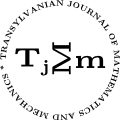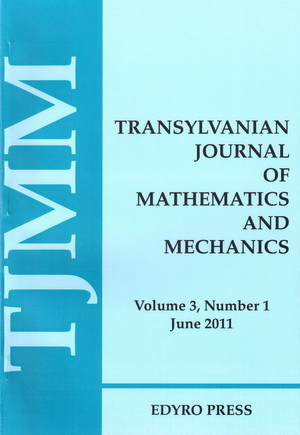ISSN: 2067-239X
ISSN(on-line): 2067-239X

Indexed in:
Mathematical Reviews
Zentralblatt MATH
EBSCO

Front cover# Volume 6 (2014), Number 2

## Hahn’s Problem with Respect to a Third-order Differential Operator

Abstract: In the present work, we are interested to the lowering operator ${𝒪}_{c;1,3,2}$, given by a Linear combination of three successive Laguerre derivatives ${𝒪}_{c;1,3,2}:={\mathsc{ℒ}}_{2,c}+3{\mathsc{ℒ}}_{1,c}+2{\mathsc{ℒ}}_{0,c},$ where ${\mathsc{ℒ}}_{i,c}:=D\left(x-c\right)D\dots \left(x-c\right)D,\phantom{\rule{1em}{0ex}}0\le i\le 2$,  (i.e. containing $\left(i+1\right)$ ordinary derivatives with respect to the $x$ variable), and $c$ is an arbitrary complex number. Then, we establish an intertwining relation between the operators ${𝒪}_{c;1,3,2}$ and the standard derivative $D$. Besides, an analogue to the Hahn problem for the operator ${𝒪}_{c;1,3,2}$ is studied. As a consequence, some integral relations between the corresponding polynomials are deduced. Finally, some expansions in series of Laguerre polynomials are presented.

## Integrable Solutions for Implicit Fractional Order Differential Equations

### Author(s): MOUFFAK BENCHOHRA and MOHAMMED SAID SOUID

Abstract: In this paper we study the existence of integrable solutions for initial value problem for fractional order implicit differential equations. Our results are based on Schauder’s ﬁxed point theorem and the Banach contraction principle ﬁxed point theorem.

## Some Integral Operators of Analytic Functions

Abstract: In the present paper we deﬁne two integral operators ${F}_{{\gamma }_{1},\dots ,{\lambda }_{l}}^{m,n}$ and ${G}_{{\gamma }_{1},\dots ,{\lambda }_{l}}^{m,n}$, deﬁned using the differential operator $S{R}^{m,n}$. We introduce some classes deﬁned by these operators and we investigate properties of the integral operators on these classes. Also, are obtained subordination results for functions $f\in \mathsc{𝒯}$ associated with the differential operator $S{R}^{m,n}$.

## Space and Time Quantization. Particles with Spin 0

### Author(s): LAUREAN HOMORODEAN

Abstract: The dynamical state of a particle with the spin 0 is described by a (4-dimensional) scalar wave function in the momentum representation. The time and the coordinates are associated with Hermitian linear operators acting on the wave functions. The eigenvalues and the eigenfunctions of the time and of the coordinates are determined by the wave equation. With its aid, we deﬁne the time-coordinate 4-tensor and the ”current density” 4-vector, which satisfy some ”conservation laws” and allow to write the time, the coordinates and the ”charge” as integrals of the components of these quantities in the momentum space. The expression of wave function in the second quantization leads to two kinds of particles: particles proper and antiparticles. We express the time, the coordinates and the ”charge” by creation and annihilation operators and determine their eigenvalues. Because the momentum is limited in value, the space and the time become discrete. Each position is populated with particles and antiparticles living a ﬁnite time. The changes in numbers of particles and antiparticles in different positions by creation and annihilation lead to displacement of the center of the particle system. This is interpreted as the macroscopic motion of a particle. Particularly, the strictly neutral particles are discussed.

## Space and Time Quantization. Particles with Spin 1/2

### Author(s): LAUREAN HOMORODEAN

Abstract: The dynamical state of a particle with the spin 1/2 is described by a (4-dimensional) bispinor wave function in the momentum representation. The time and the coordinates are associated with Hermitian linear operators acting on the wave functions. The eigenvalues and the eigenfunctions of the time and of the coordinates are determined by the wave equation expressed in diverse forms. With its aid, we deﬁne the time, the coordinates and the “charge” of the particle ﬁeld. The expression of wave function in the second quantization leads to two kinds of particles: particles proper and antiparticles. We express the time, the coordinates and the “charge” by creation and annihilation operators and determine their eigenvalues. Because the momentum is limited in value, the space and the time become discrete. Each position is populated with particles and antiparticles living a ﬁnite time. The changes in numbers of particles and antiparticles in diﬀerent positions by creation and annihilation lead to displacement of the center of the particle system. This is interpreted as the macroscopic motion of a particle.

## New Ostrowski Type Inequalities for Co-ordinated $\left(\alpha ,m\right)$ -Convex Functions

Abstract: In this paper, we establish some new Ostrowski type inequalities for functions of two variables whose derivatives in absolute value are co-ordinated $\left(\alpha ,m\right)$-convex.

## Positive Periodic Solutions for First-Order Nonlinear Neutral Functional Differential Equations with Periodic Delay

### Author(s): MOUATAZ BILLAH MESMOULI, ABDELOUAHEB ARDJOUNI and AHCENE DJOUDI

Abstract: In this paper, we study the existence of positive periodic solutions of two classes for first-order nonlinear neutral functional differential equations with periodic delay. The main tool employed here is the Krasnoselskii’s hybrid ﬁxed point theorem dealing with a sum of two mappings, one is a contraction and the other is compact. Two examples are included to illustrate our results. The results obtained here generalize the work .

## New Class of Integral Operators Preserving Subordination and Superordination for Analytic Meromorphic Functions

### Author(s): AABED MOHAMMED and MASLINA DARUS

Abstract: A certain class of integral operators ${\mathsc{𝒥}}_{\beta ,\gamma }\left(f\right)$, $\gamma ,\beta \in ℂ$, $\beta \in ℂ\setminus \left\{0\right\}$ and $\Re \left(\gamma -\beta \right)>1∕2$, of meromorphic functions in the punctured open unit disk is introduced. The main object of this paper is to investigate some subordination and superordination preserving properties of these integral operators with the sandwich type theorem.

## Hermite-Hadamard Inequalities for Modiﬁed $h$ -convex Functions

### Author(s): MUHAMMAD ASLAM NOOR, KHALIDA INAYAT NOOR and MUHAMMAD UZAIR AWAN

Abstract: In this paper, we consider the class of modiﬁed $h$ -convex functions, which was introduced by Toader . We derive Hermite-Hadamard type inequalities for the modiﬁed $h$-convex functions. Some special cases are also discussed. We try to show that this class enjoys some nice properties which the convex functions have.